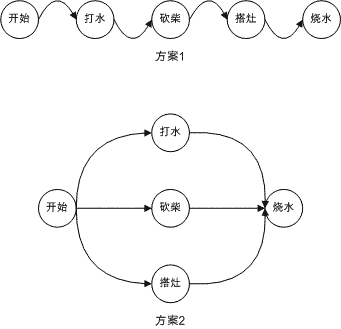# 编程之道

## 简明x86汇编语言教程（七）

### 5.0 编译优化概述

 // 程序段1int gaussianSum(){  int i, j=0;   for(i=0; i<100; i++) j+=i;  return j;}

 // 程序段1(改进1)int gaussianSum(){  int i, j=0;   for(i=1; i<100; i++) j+=i;  return j;}

 // 程序段1(改进2)inline int gaussianSum(){  return 5050;}

 // 程序段2int GetFactorial(int k){  int i, j=1;   if((k<0) || (k>=10)) return -1;  if((k<=1)) return 1  for(i=1; i

 // 程序段2 (非规范改进)int GetFactorial(int k){  int i, j=1;   static const int FractorialTable[]={1, 1, 2, 6, 24,    120, 720, 5040, 40320, 362880, 3628800};  if((k<0) || (k>=10)) return -1;  return FractorialTable[k];}

### 5.1 循环优化：强度削减和代码外提

 for(i=0; i<10; i++){  j = i;  k = j + i;}

 for(i=0; i<10; i++);j=i; k=j+i;

 j=i=10; k=20;

### 5.2 局部优化：表达式预计算和子表达式提取

 const unsigned long nGiga = 1024L * 1024L * 1024L;

 const unsigned long nGiga = 1073741824L;

 // [假设此时b, c, d, e, f, g, h都有一个确定的非零整数值，并且，// a[]为一个包括5个整数元素的数组，其下标为0到4]a = b*c;a = b+c;a = d*e;a = b*d + c*d;a = b*d*e + c*d*e;

 // [假设此时b, c, d, e, f, g, h都有一个确定的非零整数值，并且，// a[]为一个包括5个整数元素的数组，其下标为0到4]a = b*c;a = b+c;a = d*e;a = a * d;a = a * e;

 // [假设此时b, c, d, e, f, g, h都有一个确定的非零整数值，并且，// a[]为一个包括5个整数元素的数组，其下标为0到4]a = b*c;a = b+c;a = a * d;a = a * e;a = d*e;

### 5.3 全局寄存器优化

[待修订内容] 本章中，从这一节开始的所有优化都是在微观层面上的优化了。换言之，这些优化是不能使用高级语言中的对应设施进行解释的。这一部分内容将进行较大规模的修订。

 for(i=0; i<1000; i++){  for(j=0; j<1000; j++){    for(k=0; k<1000; k++){      for(l=0; l<1000; l++)        do_something(i, j, k, l);    }  }}

 int a=0; for(int i=1; i<10; i++)  for(int j=1; j<100; j++){    a += (i*j);  }

 00: 0 -> a01: 1 -> i02: 1 -> j03: i*j -> t04: a+t -> a05: j+1 -> j06: evaluate j < 10007: TRUE? goto 0308: i+1 -> i09: evaluate i < 1010: TRUE? goto 0211: [继续执行程序的其余部分]

 xor eax, eax               ; a=0(eax: a)mov edx, 1                 ; i=1(edx: i)push esi                   ; 保存esi(最后要恢复，esi作为代替j的那个循环变量)nexti:mov ecx, edx               ; [t=i]mov esi, 999               ; esi=999: 此处修改了原程序的语义，但仍为1000次循环。nextj:add eax, ecx               ; [a+=t]add ecx, edx               ; [t+=i]dec esi                    ; j--jne SHORT nextj            ; jne 等价于 jnz. [如果还需要，则再次循环]inc edx                    ; i++cmp edx, 10                ; i与10比较jl SHORT nexti             ; i < 10, 再次循环pop esi                    ; 恢复esi

### 5.4 x86体系结构上的并行最大化和指令封包CPU执行一条指令的过程基本上可以分为下面几个阶段：取指令、取数据、计算、保存数据。假设这4个阶段各需要1个时钟周期，那么，只要资源够用，并且4条指令之间不存在串行关系(换言之这些指令的执行先后次序不影响最终结果，或者，更严格地说，没有任何一条指令依赖其他指令的运算结果)指令也可以像下面这样执行：

 指令1 取指令 取数据 计　算 存数据 指令2 取指令 取数据 计　算 存数据 指令3 取指令 取数据 计　算 存数据 指令4 取指令 取数据 计　算 存数据

### 5.5 存储优化

 程序设计中的内存数据访问策略尽可能减少对于内存的访问。在不违背这一原则的前提下，如果可能，将数据一次处理完。 尽可能将数据按4或8字节对齐，以利于CPU存取 尽可能一段时间内访问范围不大的一段内存，而不同时访问大量远距离的分散数据，以利于Cache缓存*

“于是，这家伙又攒了一段代码”……

 int a[]={1,2,3,4,5,6,7,8,9,0,1,2,3,4,5,6,7,8,9,0};int i;int avg, max, min; avg=max=min=a;for(i=1; i<(sizeof(a)/sizeof(int)); i++){  avg+=a[i];  if(max < a[i])    max = a[i];  elseif(min > a[i])    min = a[i];}avg /= i;

Visual C++编译器把最开始一段赋值语句翻译成了一段简直可以说是匪夷所思的代码：

 ; int a[]={1,2,3,4,5,6,7,8,9,0,1,2,3,4,5,6,7,8,9,0};mov edi, 2                         ; 此时edi没有意义mov esi, 3                         ; esi也是！临时变量而已。mov DWORD PTR _a\$[esp+92], edimov edx, 5                         ; 黑名单加上edxmov eax, 7                         ; eax也别跑:)mov DWORD PTR _a\$[esp+132], edimov ecx, 9                         ; 就差你了，ecx; int i;; int avg, max, min;; avg=max=min=a;mov edi, 1                         ; edi摇身一变，现在它是min了。mov DWORD PTR _a\$[esp+96], esimov DWORD PTR _a\$[esp+104], edxmov DWORD PTR _a\$[esp+112], eaxmov DWORD PTR _a\$[esp+136], esimov DWORD PTR _a\$[esp+144], edxmov DWORD PTR _a\$[esp+152], eaxmov DWORD PTR _a\$[esp+88], 1       ; 编译器失误? 此处edi应更好mov DWORD PTR _a\$[esp+100], 4mov DWORD PTR _a\$[esp+108], 6mov DWORD PTR _a\$[esp+116], 8mov DWORD PTR _a\$[esp+120], ecxmov DWORD PTR _a\$[esp+124], 0mov DWORD PTR _a\$[esp+128], 1mov DWORD PTR _a\$[esp+140], 4mov DWORD PTR _a\$[esp+148], 6mov DWORD PTR _a\$[esp+156], 8mov DWORD PTR _a\$[esp+160], ecxmov DWORD PTR _a\$[esp+164], 0mov edx, edi                       ; edx是max。mov eax, edi                       ; 期待已久的avg, 它被指定为eax

 ; for(i=1; ...mov esi, edifor_loop:; avg+=a[i];mov ecx, DWORD PTR _a\$[esp+esi*4+88]add eax, ecx; if(max < a[i])cmp edx, ecxjge SHORT elseif_min; max = a[i];mov edx, ecx; else if(min > a[i])jmp SHORT elseif_minelseif_min:cmp edi, ecxjle SHORT elseif_end; min = a[i];mov edi, ecxelseif_end:; [for i=1]; i<20; i++){inc esicmp esi, 20jl SHORT for_loop; }; avg /= i;cdqidiv esi ; esi: i; ecx: 暂存变量, =a[i]; eax: avg; edx: max; 有趣的代码...并不是所有的时候都有用; 但是也别随便删除; edi: min; i++; i与20比较; avg /= i

 计时测试需要注意的问题测试必须在没有额外负荷的机器上完成。例如，专门用于编写和调试程序的计算机 尽量终止计算机上运行的所有服务，特别是杀毒程序 切断计算机的网络，这样网络的影响会消失 将进程优先级调高。对于Windows系统来说，把进程(线程)设置为Time-Critical; 对于*nix系统来说，把进程设置为实时进程 将测试函数运行尽可能多次运行，如10000000次，这样能够减少由于进城切换而造成的偶然误差 最后，如果可能的话，把函数放到单进程的系统(例如FreeDOS)中运行。

posted on 2006-11-06 10:39 编程之道 阅读(208) 评论(0)  编辑 收藏 引用 所属分类: 开发相关ASM